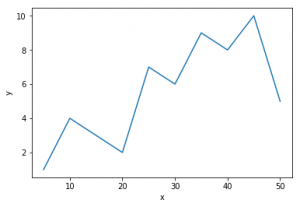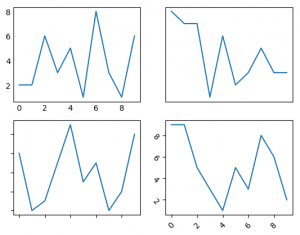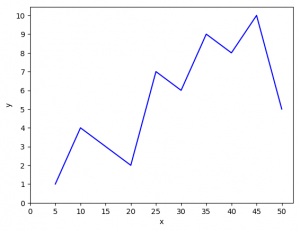# Python – Matplotlib.pyplot ticks

## Python | Matplotlib.pyplot ticks

Matplotlib is an amazing visualization library in Python for 2D plots of arrays. Matplotlib is a multi-platform data visualization library built on NumPy arrays and designed to work with the broader SciPy stack. It was introduced by John Hunter in the year 2003.
One of the greatest benefits of visualization is that it allows us visual access to huge amounts of data in easily digestible visuals. Matplotlib consists of several plots like line, bar, scatter, histogram etc.
Ticks are the values used to show specific points on the coordinate axis. It can be a number or a string. Whenever we plot a graph, the axes adjust and take the default ticks. Matplotlib’s default ticks are generally sufficient in common situations but are in no way optimal for every plot. Here, we will see how to customize these ticks as per our need.

Parameters :

Example #1: Default plot

 `# importing required modules` `import` `matplotlib.pyplot as plt` `# values of x and y axes` `x ``=` `[``5``, ``10``, ``15``, ``20``, ``25``, ``30``, ``35``, ``40``, ``45``, ``50``]` `y ``=` `[``1``, ``4``, ``3``, ``2``, ``7``, ``6``, ``9``, ``8``, ``10``, ``5``]` `plt.plot(x, y)` `plt.xlabel(``'x'``)` `plt.ylabel(``'y'``)` `plt.show()`

Output :Example #2: Playing with the ticks
Suppose we don’t want to display the values of ticks or want our ticks to be tilted or want any other customization. We can do it this way.

 `# importing libraries` `import` `random` `import` `matplotlib.pyplot as plt` `fig ``=` `plt.figure()` `# function to get random values for graph` `def` `get_graphs():` `    ``xs ``=``[]` `    ``ys ``=``[]` `    ``for` `i ``in` `range``(``10``):` `        ``xs.append(i)` `        ``ys.append(random.randrange(``10``))` `    ``return` `xs, ys` `# defining subplots` `ax1 ``=` `fig.add_subplot(``221``)` `ax2 ``=` `fig.add_subplot(``222``)` `ax3 ``=` `fig.add_subplot(``223``)` `ax4 ``=` `fig.add_subplot(``224``)` `# hiding the marker on axis` `x, y ``=` `get_graphs()` `ax1.plot(x, y)` `ax1.tick_params(axis ``=``'both'``, which ``=``'both'``, length ``=` `0``)` `# One can also change marker length` `# by setting (length = any float value)` `# hiding the ticks and markers` `x, y ``=` `get_graphs()` `ax2.plot(x, y)` `ax2.axes.get_xaxis().set_visible(``False``)` `ax2.axes.get_yaxis().set_visible(``False``)` `# hiding the values and displaying the marker` `x, y ``=` `get_graphs()` `ax3.plot(x, y)` `ax3.yaxis.set_major_formatter(plt.NullFormatter())` `ax3.xaxis.set_major_formatter(plt.NullFormatter())` `# tilting the ticks (usually needed when` `# the ticks are densely populated)` `x, y ``=` `get_graphs()` `ax4.plot(x, y)` `ax4.tick_params(axis ``=``'x'``, rotation ``=` `45``)` `ax4.tick_params(axis ``=``'y'``, rotation ``=``-``45``)` `    ` `plt.show()`

Output:Example #3: Changing the values of ticks.
In the first example, the x-axis and y-axis were divided by the value of 10 and 2 respectively. Let’s make it 5 and 1.

 `# importing libraries` `import` `matplotlib.pyplot as plt` `import` `numpy as np` `# values of x and y axes` `x ``=` `[``5``, ``10``, ``15``, ``20``, ``25``, ``30``, ``35``, ``40``, ``45``, ``50``]` `y ``=` `[``1``, ``4``, ``3``, ``2``, ``7``, ``6``, ``9``, ``8``, ``10``, ``5``]` `plt.plot(x, y, ``'b'``)` `plt.xlabel(``'x'``)` `plt.ylabel(``'y'``)` `# 0 is the initial value, 51 is the final value` `# (last value is not taken) and 5 is the difference` `# of values between two consecutive ticks` `plt.xticks(np.arange(``0``, ``51``, ``5``))` `plt.yticks(np.arange(``0``, ``11``, ``1``))` `plt.show()`

Output:The main difference from the 1st example is :
plt.xticks(np.arange(0, 51, 5))
plt.yticks(np.arange(0, 11, 1))
Changing the values in np.arange will change the range of ticks.

Reference: Matplotlib ticks.

Last Updated on November 13, 2021 by admin

## Python – Get the first key in dictionaryPython – Get the first key in dictionary

Python | Get the first key in dictionary Many times, while working with Python, we

## Difference between List and Array in PythonDifference between List and Array in Python

Difference between List and Array in Python List: A list in Python is a collection

## Generating random number list in PythonGenerating random number list in Python

Generating random number list in Python Sometimes, in making programs for gaming or gambling, we

## Get column index from column name of a given Pandas DataFrameGet column index from column name of a given Pandas DataFrame

Get column index from column name of a given Pandas DataFrame   In this article

## Deque in PythonDeque in Python

Deque in Python Deque (Doubly Ended Queue) in Python is implemented using the module “collections“.

## Pattern matching in Python with RegexPattern matching in Python with Regex

Pattern matching in Python with Regex You may be familiar with searching for text by

## Get Current Date and Time using PythonGet Current Date and Time using Python

Get Current Date and Time using Python In this example, we will learn How to

## Draw Circle in Python using TurtleDraw Circle in Python using Turtle

Draw Circle in Python using Turtle Turtle is a Python feature like a drawing board,